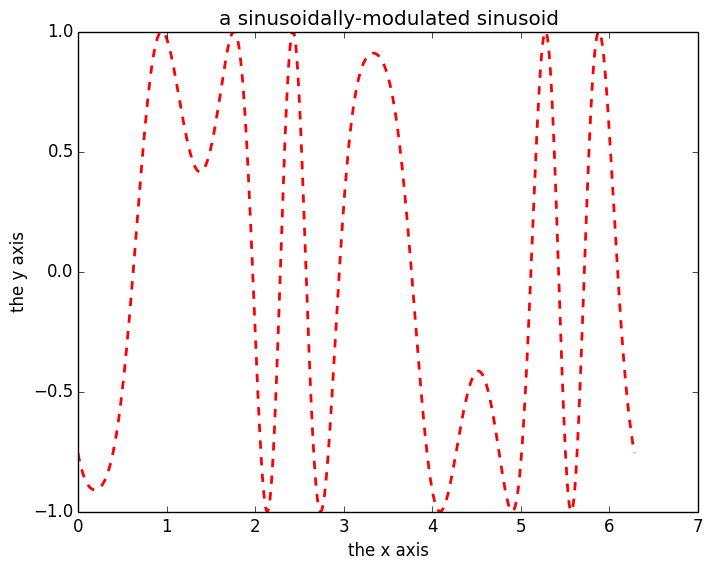# An IJulia Preview¶

This notebook is a preview demo of IJulia: a Julia-language backend combined with the IPython interactive environment. This combination allows you to interact with the Julia language using IPython's powerful graphical notebook, which combines code, formatted text, math, and multimedia in a single document.

• Note: this is a preview, because it relies on pre-release bleeding-edge versions of Julia, IPython, and several Julia packages, as explained on the IJulia github page, and functionality is evolving rapidly. We hope to have a more polished release soon.

## Basic Julia interaction¶

Basic mathematical expressions work like you expect:

In :
function f(x)
y = x.^2;
return y;
end
g = x -> x.^2

Out:
(anonymous function)
In :
a = randn(3,3);
f(a) - g(a)

Out:
3x3 Array{Float64,2}:
0.0  0.0  0.0
0.0  0.0  0.0
0.0  0.0  0.0
In :
a[:]

Out:
9-element Array{Float64,1}:
-0.112644
-0.772488
0.172679
-0.0922463
0.295773
0.73591
0.172949
0.797139
1.32277  

You can define variables, write loops, and execute arbitrary multiline code blocks. Here is an example of an alternating harmonic series $\sum_{n=1}^\infty \frac{(-1)^n}{n}$ from a Julia tutorial by Homer Reid:

In :
s = 0.0
for n = 1:2:10000
s += 1/n - 1/(n+1)
end
s # an expression on the last line (if it doesn't end with ";") is printed as "Out"

Out:
0.6930971830599458

Previous outputs can be referred to via Out[n], following the IPython, for example Out for the result above. You can also use the shorthand _2, or _ for the previous result, as in IPython. Like in Matlab, ans can also be used to refer to the previous result, even if it was not printed (when the command ended with ;).

For example, the harmonic series above should be converging (slowly) to $\ln 2$, and we can check this:

In :
Out - log(2)

Out:
-4.9997499999454575e-5

Like Matlab or Scipy + Numpy, Julia has lots of mathematical functions and linear algebra built in. For example, we can define a $500\times500$ random matrix $R$ and form the positive-definite matrix $R^* R$:

In :
R = rand(500,500)
R' * R  + 1

Out:
500x500 Array{Float64,2}:
176.823  134.171  133.605  128.97   …  131.832  140.237  128.797  130.203
134.171  178.431  133.187  130.039     130.756  139.909  133.399  133.329
133.605  133.187  175.321  126.044     127.895  139.384  128.22   127.631
128.97   130.039  126.044  167.221     122.407  134.698  125.822  126.318
131.108  129.587  129.609  125.715     128.892  136.986  130.606  125.784
124.509  128.229  127.388  120.704  …  127.665  133.017  123.582  122.957
139.466  134.465  134.819  131.503     129.889  142.04   133.791  130.107
127.433  128.528  126.477  121.89      125.627  129.983  126.212  122.738
129.841  134.427  129.799  124.671     127.271  135.654  124.512  125.128
136.525  136.118  131.637  126.53      130.56   138.127  131.584  131.009
127.526  132.144  124.117  128.28   …  125.29   137.683  128.406  127.502
128.645  132.295  126.274  119.687     123.766  136.288  124.935  124.505
136.68   135.109  131.409  128.654     128.63   138.816  129.705  128.02
⋮                                 ⋱
130.795  138.867  132.224  128.122     126.996  138.34   126.832  129.769
129.789  129.394  126.042  123.559     127.461  134.27   128.887  122.782
126.268  124.795  121.26   122.385  …  125.079  130.908  121.051  121.702
134.162  134.784  132.64   125.757     125.919  135.867  131.054  129.26
120.942  132.027  127.296  119.716     123.719  131.701  125.041  125.271
130.304  128.282  126.602  125.55      122.287  136.835  125.922  123.306
136.932  135.336  134.88   134.972     133.228  141.934  133.944  133.537
129.669  135.645  129.288  128.062  …  127.752  137.66   132.081  126.145
131.832  130.756  127.895  122.407     163.939  134.377  124.911  124.837
140.237  139.909  139.384  134.698     134.377  189.742  134.529  135.962
128.797  133.399  128.22   125.822     124.911  134.529  171.358  128.957
130.203  133.329  127.631  126.318     124.837  135.962  128.957  165.798

(Notice that, by default, only a portion of a large matrix is shown. You didn't really want to read $500^2 = 250,000$ numbers, did you?)

Standard output from Julia is also captured and sent to the IJulia notebook as you'd expect:

In :
println("Hello world!\n")
println(STDERR, "Börk börk börk, some unicode output on stderr...\n")

Hello world!


Börk börk börk, some unicode output on stderr...



IJulia even captures output from external C libraries (and notice how easy it is using Julia's ccall intrinsic):

In :
ccall(:printf, Cint, (Ptr{Uint8},), "Hello from C!!\n");

Hello from C!!


We can define functions, of course, and use them in later input cells:

In :
f(x) = x + 1

Out:
f (generic function with 1 method)
In :
println(f(3))
f([1,1,2,3,5,8])

4

Out:
6-element Array{Int64,1}:
2
2
3
4
6
9

Notice that the input above both printed an scalar to STDOUT and also returned a vector, the latter using Julia's ability to write polymorphic functions and built-in array operations.

On the other hand adding a string to a number is not defined (there is no + method defined for those types, although we could easily add one), and attempting to do so will throw an exception:

In :
f("Hello?")

+ has no method matching +(::ASCIIString, ::Int64)

in f at In:1

## Julia–Python interoperability: SciPy and Matplotlib¶

Julia can easily and transparently call external Python code using a package called PyCall, and to illustrate that capability we will show some examples calling SciPy and Matplotlib from Julia.

For example, we can use the Newton solver in scipy.optimize to solve a transcendental equation $\cos(x) - x = 0$ given a Julia function:

In :
using PyCall
@pyimport scipy.optimize as so
so.newton(x -> cos(x) - x, 1)

Out:
0.7390851332151607

We can use the same @pyimport syntax to import Matplotlib (specifically, the matplotlib.pyplot module), but to integrate Matplotlib's graphics with the IJulia display requires a little more work. To simplify this, we've created a PyPlot module for Julia:

In :
using PyPlot
x = linspace(0,2*pi,1000)
y = sin(3*x + 4*cos(2*x))
plot(x, y, color="red", linewidth=2.0, linestyle="--")
ylabel("the y axis")
xlabel("the x axis")
title("a sinusoidally-modulated sinusoid")

INFO: Loading help data...Out:
PyObject <matplotlib.text.Text object at 0x122e1cb90>
In :
g = imread("nt_toolbox/data/hibiscus.bmp");

In :
imshow(g, interpolation="nearest")
set_cmap("gray")
axis("off")Out:
(-0.5,511.5,511.5,-0.5)
In :
include("nt_toolbox/nt_signal.jl")
using nt_signal
imageplot(g)

Warning: replacing module nt_signal